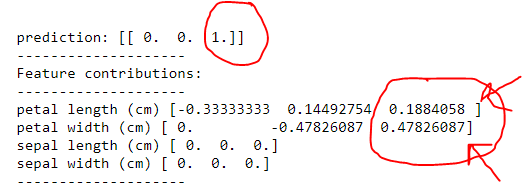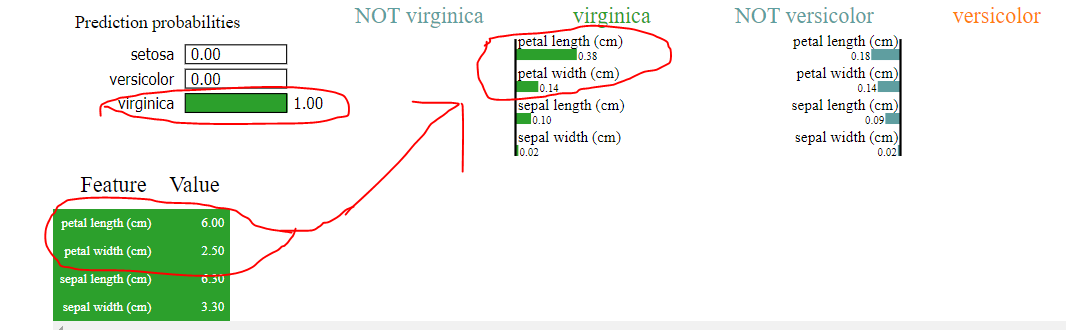2 views

Lime source: https://github.com/marcotcr/lime

treeinterpreter source: tree interpreter

I am trying to understand how the decision tree made its predictions using Lime and tree interpreter. While both claim they are able to interpret the decision tree in their description. It seems like both interpret the same DecisionTree in different ways. That is the feature contribution order. How is that possible? if both are looking at the same thing and are trying to describe the same event but assign importance in a different order.

Who should we trust? Especially where the top feature does matter in prediction.

The code for tree

import sklearn

import sklearn.datasets

import sklearn.ensemble

import numpy as np

import lime

import lime.lime_tabular

from __future__ import print_function

np.random.seed(1)

from treeinterpreter import treeinterpreter as ti

from sklearn.tree import DecisionTreeClassifier

dt = DecisionTreeClassifier(random_state=42)

dt.fit(iris.data, iris.target)

n = 100

instances =iris.data[n].reshape(1,-1)

prediction, biases, contributions = ti.predict(dt, instances)

for i in range(len(instances)):

print ("prediction:",prediction)

print ("-"*20)

print ("Feature contributions:")

print ("-"*20)

for c, feature in sorted(zip(contributions[i],

iris.feature_names),

key=lambda x: ~abs(x.any())):

print (feature, c)

The code for lime

import sklearn

import sklearn.datasets

import sklearn.ensemble

import numpy as np

import lime

import lime.lime_tabular

from __future__ import print_function

np.random.seed(1)

from sklearn.tree import DecisionTreeClassifier

dt = DecisionTreeClassifier(random_state=42)

dt.fit(iris.data, iris.target)

explainer = lime.lime_tabular.LimeTabularExplainer(iris.data, feature_names=iris.feature_names,

class_names=iris.target_names,

discretize_continuous=False)

n = 100

exp = explainer.explain_instance(iris.data[n], dt.predict_proba, num_features=4, top_labels=2)

exp.show_in_notebook(show_table=True,  predict_proba= True , show_predicted_value = True , show_all=False)

Let's look first at the output of the tree.so it did correctly say it was a virginica. However by assigning the importance in

1) petal width (cm) then petal length (cm)

Now let's look at the output of limeYes, it does say the algorithm predicted virginica however looking at how it made that classification, we clearly see the following

1) petal length (cm) > petal width (cm) in lime instead of petal length (cm) < petal width (cm) as shown in tree

2) where sepal width and sepal length was predicted zero, lime claims of a certain value, as shown in the uploaded images

What is happening here?

The problem grows when the features are 1000+ where every digit does matter to take a decision.

by (33.1k points)

Lime: It is used for local linear approximation of the model's behavior. This model may be quite complex globally, it is easier to approximate it around the area of a particular instance. While treating the model as a black box, we stuff the instance we want to explain and learn a sparse linear model around it. The model's decision function is described by the blue/pink background and is clearly nonlinear. The bright red cross is the instance being explained (let's call it X). We sample instances around X and weight them according to their proximity to X (weight here is indicated by size). We then learn a linear model (dashed line) that approximates the model well in the vicinity of X, but not necessarily globally.

For example:

Let's say we have a node that compares feature F to some value and splits instances based on that. Suppose that 50% of all instances reaching that node refer to class C. Suppose we have a new instance, and it ends up getting attached to the left child of that node, now 80% of all instances belong to class C. Then, the contribution of feature F for this decision is computed as 0.8 - 0.5 = 0.3

Comparison: Lime is a model-independent method, which is based on local linear approximation. Treeinterpreter operates in a similar manner to the Decision Tree itself, and really look at which features are actually used in comparisons by the algorithm. So they're really fundamentally doing quite different things.